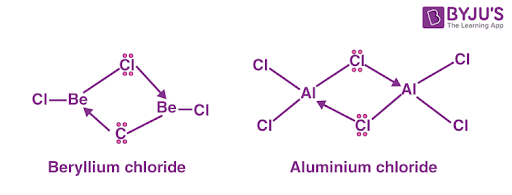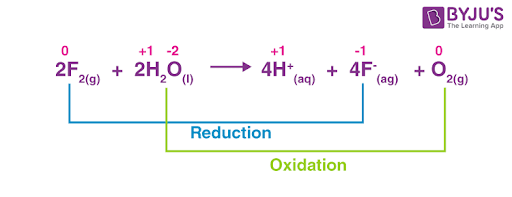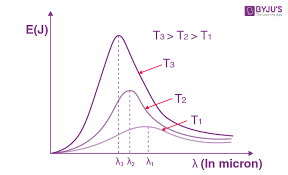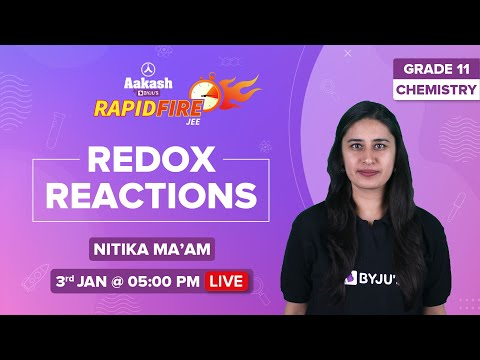# Important 2 Marks Questions for CBSE 11 Chemistry

Class 11 chemistry important 2 mark questions with answers are provided here. These important questions are based on CBSE board curriculum and correspond to the most recent Class 11 chemistry syllabus. By practicing these Class 11 important 2 mark questions, students will be able to quickly review all of the ideas covered in the chapter and prepare for the Class 11 Annual examinations as well as other entrance exams such as NEET and JEE.

Download Class 11 Chemistry Important 2 Mark Questions with Answers PDF by clicking on the button below.

## Class 11 Chemistry Important 2 Mark Questions with Answers

Q1:

Why does the rainwater normally have a pH of about 5.6? When does it become acid rain?

Rainwater is acidic, with a pH of about 5.6. This is due to CO2 being dissolved in the water vapours present, resulting in acidic H2CO3. Acid rain occurs when the pH of rainwater drops to around 3 to 4.5 due to the breakdown of nitrogen and sulphur oxides in the water.

Q2:

A compound C7H14 on ozonolysis gives ethanal and pentan–3- one. What is the structure of alkene?

3-Ethylpent-2-ene is the alkene. It produces a combination of ethanal and pentan-3-one after ozonolysis.

Q3:

A metal ‘X’ is present in chlorophyll. Identify the metal ‘X’. How does this metal react with N2?

Magnesium is the metal – X found in chlorophyll. Magnesium nitride is formed when magnesium combines with nitrogen gas.

$$\begin{array}{l}3Mg+N2\to Mg_{3}N_{2}\end{array}$$

Q4:

An element ‘A’ belongs to group 2 of the periodic table. It shows anomalous behaviour from the rest of the elements of its group. It shows a diagonal relationship with another element, ‘B’. Chlorides of both ‘A’ and ‘B’ have a bridged structure in the vapour phase. Identify A and B and draw the structures of their respective chlorides.

Element A is Beryllium, and element B is Aluminium.Q5:

Consider the reaction of water with F2 and suggest, in terms of oxidation and reduction, which species are oxidised/ reduced.

$$\begin{array}{l}2F_{2(g)} + 2H_{2}O_{(l)} \to 4H^{+}_{(aq)} + 4F^{-}_{(aq)} + O_{2(g)}\end{array}$$

Water is oxidised to oxygen, while fluorine is reduced to fluoride ion, an example of a redox reaction. Various species’ oxidation numbers can be represented as:The oxidation state of fluorine is decreased from zero to (– 1). A drop in the oxidation state shows fluorine reduction.

Water is oxidised from the oxidation state of (– 2) to the oxidation state of zero. An increase in oxidation state indicates water oxidation.

Q6:

The critical temperature of ammonia and carbon dioxide is 405.5 K and 304.10 K, respectively. Which of these gases will liquefy first when you start cooling from 500K to their critical temperature?

The critical temperature is when no amount of pressure will cause gases to liquefy. Because its critical temperature is reached first, ammonia will liquefy first.

Q7:

The drain cleaner contains small bits of aluminium that react with caustic soda to produce dihydrogen gas. What volume of dihydrogen at 20°C and one bar pressure will be released when 0.15 g of aluminium reacts?

Dihydrogen is formed when Al reacts with caustic soda; the reaction is going to be

$$\begin{array}{l}2Al + 2NaOH + 2H_{2}O \to 2NaAlO_{2} + 3H_{2}\end{array}$$

So we see that 2 mol of Al produces 3 moles of H2

∴ 2 × 27 g of Al produce 3 × 2g of H2.

∴ 54 g of Al produces 6g of H2.

∴ 1g of Al produces 6/54 = 1/9 g of H2.

∴ 0.15g of Al produces 0.15/9 g of H2

So, the number of moles of H2 produce = weight of HE produce/molar weight of H2

= (0.15/9)/2

= 0.15/18 = 0.00833 mol

Now, using formula, PV = nRT

Here, P = 1 bar or 0.987 atm

T = 20°C or 293K

n = 0.00833 mol

R = 0.0821 atm dm³/K/mol

V = ?

V = 0.00833 × 0.0821 × 293/0.987

= 0.203 dm³

We know, 1 dm³ = 1000 mL

so, Volume of H2 = 1000× 0.203mL = 203mL

Q8:

(a) Write the atomic number of the element present in the third period and seventeenth group of the periodic table.

(b) Out of the elements Cr (Z = 24), Mg (Z=12) and Fe (Z =26), identify the element with five electrons in the 3d subshell.

Because the third period (n = 3) begins with Z = 11 and finishes with Z = 18, the element is chlorine (CI) with atomic number (Z) = 17, which is found in the periodic table’s third period and seventeenth group.

Electronic configuration of Cr = [Ar] 3d54s1

Electronic configuration of Mg = [Ne] 3s2

Electronic configuration of Fe = [Ar] 3d6 4s2

Thus, Cr (Z=24) has five electrons in a three-dimensional orbit.

Q9:

Which of the following statements related to the modern periodic table is incorrect and why?

(a) Each block contains a number of columns equal to the number of electrons that can occupy that subshell.

(b) The d – block has 8 columns because a maximum of 8 electrons can occupy all the orbitals in the d – subshell.

Statement (b) is incorrect.

Because a maximum of 10 electrons can fill all of the orbitals in a d subshell, the d-block contains ten columns rather than eight.

Q10:

Among the elements B, Al, C and Si:

(a) Which has the highest first ionization enthalpy?

(b) Which has the most negative electron gain enthalpy? Give reason

(a)

Carbon has the highest initial ionisation enthalpy amongst B, Al, C, and Si. Because of the higher nuclear charge, C has a higher initial ionisation enthalpy than B. B and Al are members of the same group, and because B is smaller, it has a greater value than Al. C and Si are also members of the same group. However, C has a greater value than Si due to its smaller size. The first ionisation enthalpy of these elements is in the following order: Al < Si < B < C.

(b)

Aluminium is the most metallic among the elements B, Al, C, and Si. Metallic nature reduces throughout time yet increases from top to bottom in a group.

Q11:

What is the shielding effect? How does it affect atomic size in lanthanides?

The shielding effect is defined as the phenomenon in which the inner-shell electrons buffer the outer-shell electrons from the nuclear charge. The Lanthanide Contraction is caused by the 4f electrons’ weak shielding effect.

Q12:

Find the number of moles of 4.9g of sulphuric acid?

Given,

Mass of the Sulphuric Acid (H2SO4) = 4.9 g.

Atomic weight =2+32+4×16 = 98g/mol

Now,

No. of moles of the Sulphuric Acid = Gram weight/Atomic weight

∴ No. of moles of the sulphuric acid = 4.9/98 = 0.05 moles.

Hence, the number of moles of Sulphuric Acid is 0.05 moles.

Q13:

Show how hyperconjugation occurs in propene molecules.

Hyperconjugation is a stabilising interaction that occurs when electrons in a sigma-bond (typically C-H or C-C) engage with an adjoining vacant or partially filled p-orbital or a pi-orbital, resulting in an expanded molecular orbital that boosts the system’s stability.

Hyper conjugation occurs in the propene molecule due to a partial overlap of the sp 3-s sigma bond orbital with the vacant p-orbital of a nearby carbon atom. This causes the pi-electrons to delocalize, increasing the molecule’s stability.

Q14:

How can you explain the formation of NaCl according to the Kossel concept?

According to the Kossel theory, atoms must complete their octet in order to participate in bond formation. To do so, they must first reach their closest configuration of inert gas atoms, which can be attained by gaining, losing, and sharing electrons.

After the entire transfer of electrons from one atom to another, a chemical connection is created, and the atoms attain the closest configuration of a noble gas.

The formation of NaCl can be explained as:

$$\begin{array}{l}Na \to Na^{+}+e^{-}\end{array}$$
$$\begin{array}{l}[Ne] 3s^{1}\to [Ne]\end{array}$$
$$\begin{array}{l}Cl + e^{-}\to Cl^{-}\end{array}$$
$$\begin{array}{l}[Ne]3s^{2}3p^{5} . [Ne]3s^{2}3p^{6} or [Ar]\end{array}$$
$$\begin{array}{l}Na^{+}+Cl^{-}\to Na^{+}Cl^{-} or NaCl\end{array}$$

Q15:

What is a black body? What are the characteristics of black body radiation?

A blackbody is an item that absorbs all radiation, including visible light, that strikes it.

Black body radiation is the radiation generated by a black body. The image depicts the energy distribution of black body radiation at various temperatures and wavelengths.Black body radiation’s characteristics are as follows:

• A black body emits radiation in the ultraviolet, visible light, and infrared wavelength ranges.
• Stefan’s law states that as the temperature of a black body rises, so does the total amount of radiation it emits.
• The distribution of energy is not even. At a wavelength of λm, the amount of energy emitted is at its highest.
• With increasing temperature, the wavelength λm for which the energy emitted is highest decreases.

Q16:

Why is Ga smaller in size than Al?

Because there are 10 elements in the first transition series between Ga and Al that have electrons in the inner d-orbital, Ga’s atomic radius is less than Al’s. Due to their structure and low penetrating capability, the inner d-orbitals are ineffective at shielding the nucleus. As a result, Ga’s effective nuclear charge is more than Al’s, and Ga’s atomic radius is less than Al’s.

Q17:

Express 32.392800 to four significant figures

After rounding off up to 4 significant figures, we get 32.39.

Q18:

If a tank is full of water coming in and out at the same rate, then what will happen to the level of water in a tank?

The water level will remain constant.

Q19:

Why should the bottle containing hydrogen peroxide be cooled before opening?

This is because hydrogen peroxide decomposes into water or oxygen when heated or at a little higher temperature.

Q20:

Why the symbols of

$$\begin{array}{l}_{79}^{35}\textrm{Br}\end{array}$$
and
$$\begin{array}{l}_{}^{35}\textrm{Br}\end{array}$$
are not acceptable?

The number of protons in each isotope of a given element is the same, although the mass number for the same atomic number can vary. As a result, the mass number must be written down.

Because the atomic number of Br cannot reach 79 (greater than the mass number of 35), the symbol

$$\begin{array}{l}_{79}^{35}\textrm{Br}\end{array}$$
is not approved. Similarly, the symbol
$$\begin{array}{l}_{}^{35}\textrm{Br}\end{array}$$
is not acceptable because the mass number must be stated. This is required to distinguish between an element’s isotopes.

## Redox Reactions MCQsTest your Knowledge on Important 2 marks questions for cbse class 11 chemistry!